# 平移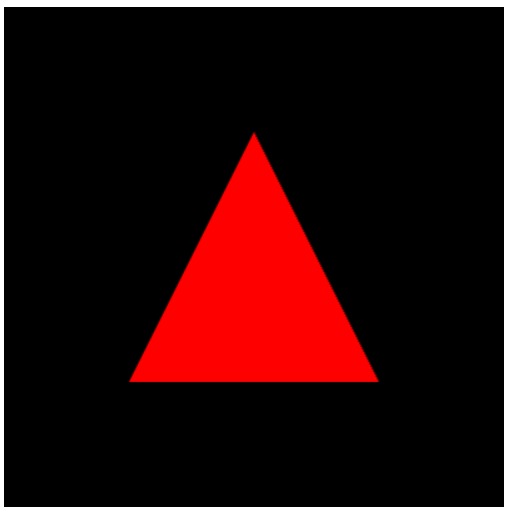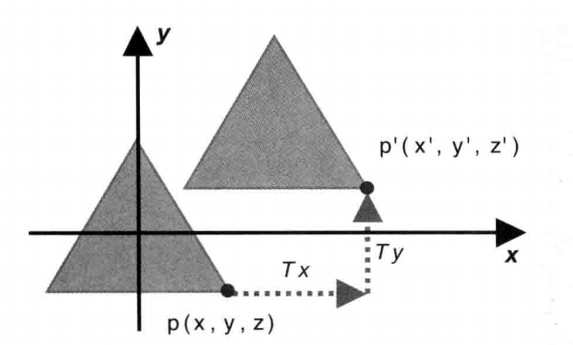// 顶点着色器代码(决定顶点的位置、大小、颜色)
'attribute vec4 a_Position;\n' +
'uniform vec4 u_Translation;\n' +
'void main() {\n' +
'  gl_Position = a_Position + u_Translation;\n' + // 设置顶点的位置
'  gl_PointSize = 10.0;\n' +      // 设置顶点的大小
'}\n';

1、添加了 uniform vec4 u_Translation 定义
2、顶点的位置使用两个 vec4 变量进行相加， gl_Position = a_Position + u_Translation

// 三角形顶点的平移量
var Tx = 0.5, Ty = 0.5, Tz = 0.0;
var u_Translation = context.getUniformLocation(context.program,'u_Translation');
context.uniform4f(u_Translation, Tx, Ty, Tz, 0.0);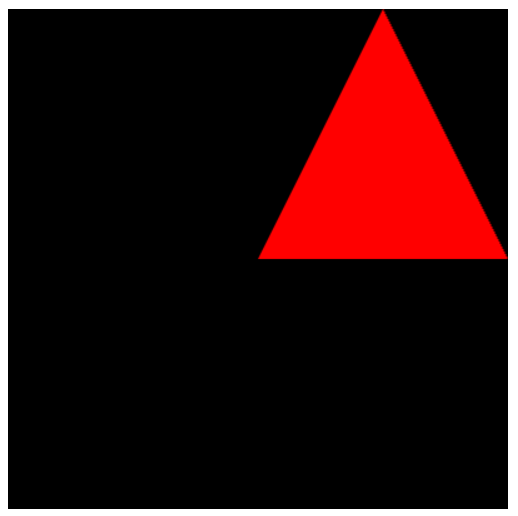# 旋转

1. 绕着哪个轴旋转
2. 旋转的方向（顺时针还是逆时针）
3. 旋转的角度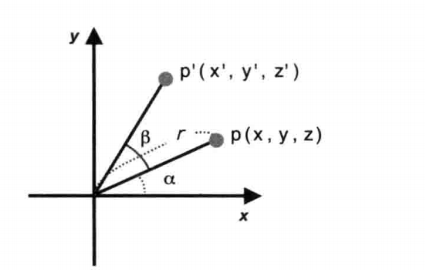x=rcosαy=rsinα

x=rcos(α+β)=r(cosαcosβsinαsinβ)y=rsin(α+β)=r(sinαcosβ+cosαsinβ)

x=xcosβysinβy=xsinβ+ycosβz=z

// 顶点着色器代码(决定顶点的位置、大小、颜色)
'attribute vec4 a_Position;\n' +
'uniform float u_CosB, u_SinB;\n' +
'void main() {\n' +
'gl_Position.x = a_Position.x * u_CosB - a_Position.y * u_SinB;\n' +
'gl_Position.y = a_Position.x * u_SinB + a_Position.y * u_CosB;\n' +
'gl_Position.z = a_Position.z;\n' +
'gl_Position.w = 1.0;\n' +
'gl_PointSize = 10.0;\n' +      // 设置顶点的大小
'}\n';

// 旋转的角度
var ANGLE = 30;
var radian = Math.PI * ANGLE / 180.0; //转为弧度

var u_CosB = context.getUniformLocation(context.program, 'u_CosB');
var u_SinB = context.getUniformLocation(context.program, 'u_SinB');
context.uniform1f(u_CosB, cosB);
context.uniform1f(u_SinB, sinB);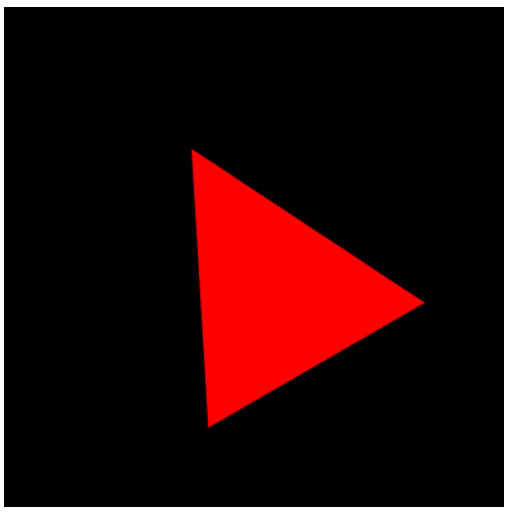# 缩放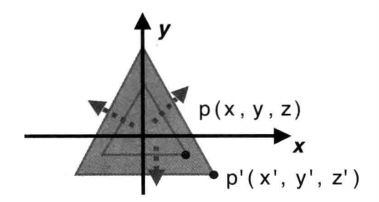p(x,y,z)$p(x,y,z)$ 缩放后，在三个轴的缩放因子为：Sx$S_x$Sy$S_y$Sz$S_z$，则缩放后，点p(x,y,z)${p'(x',y',z')}$的坐标如下：

x=xSxy=ySyz=zSz

// 顶点着色器代码(决定顶点的位置、大小、颜色)
'attribute vec4 a_Position;\n' +
'uniform float a_Scale;\n' +
'void main() {\n' +
'  gl_Position.x = a_Position.x * a_Scale;\n' +
'  gl_Position.y = a_Position.y * a_Scale;\n' +
'  gl_Position.z = a_Position.z * a_Scale;\n' +
'  gl_Position.w = 1.0;\n' +
'  gl_PointSize = 10.0;\n' +      // 设置顶点的大小
'}\n';

// 三角形顶点的缩放
var scale = 0.5;
var u_Scale = context.getUniformLocation(context.program, 'a_Scale');
context.uniform1f(u_Scale, scale);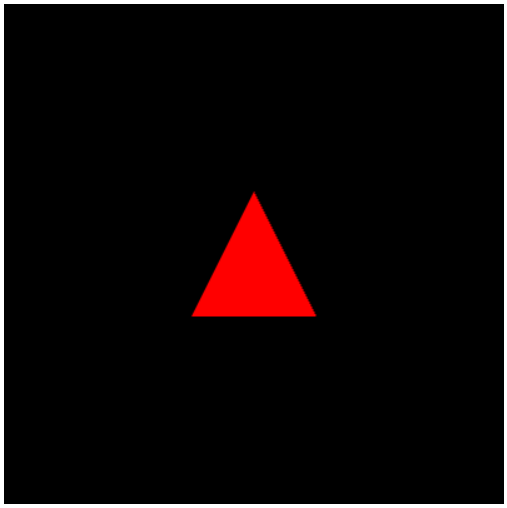# 参考

<<WebGL编程指南>>©️2019 CSDN 皮肤主题: 大白 设计师: CSDN官方博客